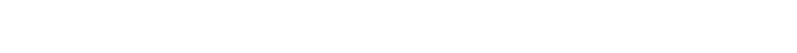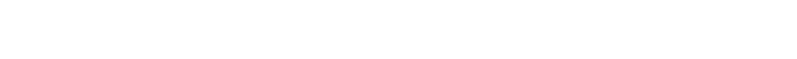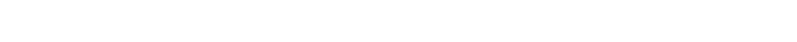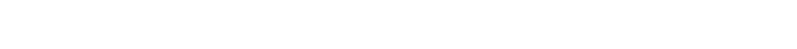## Search

Machine learning Filter

# What is K-Nearest Neighbor in Machine Learning: K-NN Algorithm

10025If you are thinking of a simple, easy-to-implement supervised machine learning algorithm which can be used to solve both classification as well as regression problems, K-Nearest Neighbor (K-NN) is the perfect choice. Learning K-NN is a great way to introduce yourself to machine learning and classification in general. Also, you will find a lot of intense application of K-NN in data mining, pattern recognition, semantic searching, intrusion detection and anomaly detection.

K-Nearest Neighbors is one of the most basic supervised machine learning algorithms, yet very essential. A supervised machine learning algorithm is one of the types of machine learning algorithm which is dependent on labelled input data in order to learn a function which is capable of producing an output whenever a new unlabeled data is given as input.

In real life scenarios, K-NN is widely used as it is non-parametric which means it does not make any underlying assumptions about the distributions of data. With the business world entirely revolving around Data Science, it has become one of the most lucrative fields. Hence, the heavy demand for a Data Science Certification.

## Parametric vs Non-parametric Methods

Let us look into how different is a parametric machine learning algorithm from a nonparametric machine learning algorithm.

Machine learning, in other words can be called as learning a function (f) which maps input variables (X) to the output variables (Y).

Y=f(X)

An algorithm learns about the target mapping function from the training data. As we are unaware of the form of the function, we have to evaluate various machine learning algorithms and figure out which algorithms perform better at providing an approximation of the underlying function.

Statistical Methods are classified on the basis of what we know about the population we are studying.

• Parametric statistics is a branch of statistics which assumes that sample data comes from a population that follows a probability distribution based on a fixed set of parameters.
• Nonparametric statistics is the branch of statistics that is not based solely on population parameters.

### Parametric Machine Learning Algorithms

This particular algorithm involves two steps:

1. Selecting a form for the function
2. Learning the coefficients for the function from the training data

Let us consider a line to understand functional form for the mapping function as it is used in linear regression and simplify the learning process.

b0 + b1*x1 + b2*x2 = 0

Where b0, b1 and b2 are the coefficients of the line which control the intercept and slope, and x1 and x2 are two input variables.

All we have to do now is to estimate the coefficients of the line equation to get a predictive model for the problem. Now, the problem is that the actual unknown underlying function may not be a linear function like a line. In that case, the approach will give poor results. Some of the examples of parametric machine learning algorithms are mentioned below:

• Logistic Regression
• Linear Discriminant Analysis
• Perceptron
• Naive Bayes
• Simple Neural Networks

### Nonparametric Machine Learning Algorithms

Nonparametric methods always try to find the best fit training data while constructing the mapping function which also allows it to fit a large number of functional forms. Some of the examples of nonparametric machine learning algorithms are mentioned below:

• k-Nearest Neighbors
• Decision Trees like CART and C4.5
• Support Vector Machines

The best example of nonparametric machine learning algorithms would be k-nearest neighbors algorithm which makes predictions based on the k most similar training patterns for a given set of new data instance. This method simply assumes that the patterns which are close are likely to be of similar type.

Parametric Machine Learning AlgorithmsNonparametric Machine Learning Algorithms
Benefits
• Simple to understand and interpret results
• Speed of learning from data in fast
• Less training data is required

• Flexible enough to fit a large number of functional forms
• No assumptions about the underlying functions
• Provides high performance for prediction

Limitations
• Choosing a functional form constrains the method to the specified form
• It has limited complexity and more suited to simpler problems
• It is unlikely to match the underlying mapping function and results in poor fit

• Requires more training data in order to estimate the mapping function
• Due to more parameters to train, it is slower comparatively
• There is a risk to overfit the training data

## Method Based LearningThere are several learning models namely:

• Association rules based
• Ensemble method based
• Deep Learning based
• Clustering method based
• Regression Analysis based
• Bayesian method based
• Dimensionality reduction based
• Kernel method based
• Instance based

Let us understand what Instance Based Learning is all about.

### Instance Based Learning (IBL)• Instance-Based methods are the simplest form of learning
• Instance -Based learning is lazy learning
• K-NN model works on identified instance
• Instances are retrieved from memory and then this data is used to classify the new query instance
• Instance based learning is also called memory-based or case-based

Under Instance-based Learning we have,

Nearest-neighbor classifier

Uses k “closest” points (nearest neighbors) for performing classification. For example: It’s how people judge by observing our peers. We tend to move with people of similar attributes.

### Lazy Learning vs Eager Learning

Lazy LearningEager Learning
Simply stores the training data and waits until it is given a test tuple.Munges the training data as soon as it receives it.
It's slow as it calculates based on the current data set instead of coming up with an algorithm based on historical data.It's fast as it has pre-calculated algorithm.
Localized data so generalization takes time at every iteration.On the basis of training set ,it constructs a classification model before receiving new data to classify.

## What is K-NN?

One of the biggest applications of K-Nearest Neighbor search is Recommender Systems. If you have noticed while you are shopping as a user on Amazon and you like a particular item, you are recommended with similar items.It also recommends similar items bought by other users and other set of items which are often bought together. Basically, the algorithm compares the set of users who like each item and looks for similarity. This not only applies to recommending items or products but also recommending media and even advertisements to display to a user.

K nearest neighbors or K-NN Algorithm is a simple algorithm which uses the entire dataset in its training phase. Whenever a prediction is required for an unseen data instance, it searches through the entire training dataset for k-most similar instances and the data with the most similar instance is finally returned as the prediction.

This algorithm suggests that if you’re similar to your neighbours, then you are one of them. Let us consider a simple example, if apple looks more similar to peach, pear, and cherry (fruits) than monkey, cat or a rat (animals), then most likely apple is a fruit.

Nearest Neighbours algorithm has been in action for the last sixty years. It is mainly used in statistical estimation and pattern recognition, as a non-parametric method, for regression and classification. The main aim of the K-Nearest Neighbor algorithm is to classify a new data point by comparing it to all previously seen data points. The classification of the k most similar previous cases are used for predicting the classification of the current data point. It is a simple algorithm which stores all available cases and classifies new cases based on a similarity measure (e.g., distance functions).

## When do we use K-NN algorithm?

K-NN algorithm can be used for applications which require high accuracy as it makes highly accurate predictions. The quality of predictions is completely dependent on the distance measure. Thus, this algorithm is suitable for applications for which you have sufficient domain knowledge so that it can help you select an appropriate measure.

As we have already seen K-NN algorithm is a type of lazy learning, the computation for the generation is postponed until classification which indeed increases the costs of computation compared to other machine learning algorithms. But still K-NN is considered to be the better choice for applications where accuracy is more important and predictions are not requested frequently.

K-NN can be used for both regression and classification predictive problems. However, in the industry it is mostly used in classification problems.

Generally we mainly look at 3 important aspects in order to evaluate any technique:

1. Ease to interpret output
2. Calculation time
3. Predictive Power

Let us consider a few examples to place K-NN in the scale :If you notice the chart mentioned above, K-NN algorithm exceeds in most of the parameters. It is most commonly used for ease of interpretation and low calculation time.

## How does the K-NN algorithm work?

K-NN algorithm works on the basis of feature similarity. The classification of a given data point is determined by how closely out-of-sample features resemble our training set.The above figure shows an example of k-NN classification. If you consider the nearest neighbor to the test sample, it is a blue square (Class 1) and k=1. This falls inside the inner circle.

Now, if you consider k=3, then you will see 2 red triangles and only 1 blue square falls under the outer circle. Thus, the test sample is classified as a red triangle now (Class 2).

Similarly, if you consider k=5, it is assigned to the first class (3 squares vs. 2 triangles outside the outer circle).

## K-NN in Regression

In regression problems, K-NN is used for prediction based on the mean or the median of the K-most similar instances.

## K-NN in Classification

K-nearest-neighbor classification was actually developed from the need to perform discriminant analysis when reliable parametric estimates of probability densities are unknown or are difficult to determine. When K-NN is used for classification, the output is easily calculated by the class having the highest frequency from the K-most similar instances. The class with maximum vote is taken into consideration for prediction.

The probabilities of Classes can be calculated as the normalized frequency of samples that belong to each class in the set of K most similar instances for a new data instance.

For example, in a binary classification problem (class is 0 or 1):

p(class=0) = count(class=0) / (count(class=0)+count(class=1))

If you are using K and you have an even number of classes (e.g. 2) it is a good idea to choose a K value with an odd number to avoid a tie. And the inverse, use an even number for K when you have an odd number of classes.

Ties can be broken consistently by expanding K by 1 and looking at the class of the next most similar instance in the training dataset.

## Making Predictions with K-NN

A case can be classified by a majority vote of its neighbors. The case is then assigned to the most common class amongst its K nearest neighbors measured by a distance function. Suppose the value of K is 1, then the case is simply assigned to the class of its nearest neighbor.The three distance measures mentioned above are valid only for continuous variables. For categorical variables, the Hamming distance is used. It also brings up the issue of standardization of the numerical variables between 0 and 1 when there is a mixture of numerical and categorical variables in the dataset.By inspecting the data, you can choose the best optimal value for K. Generally, a large value of K is more accurate as it tends to reduce the overall noise but is not always true. Another way to retrospectively determine a good K value by using an independent dataset to validate the K value is Cross-validation. According to observation, the optimal K for most datasets has been between 3-10 which provides better results than 1NN.

For example, let us consider an example where the data mentioned below us concerned with credit default. Age and Loan are two numerical variables (predictors) and Default is the target.By observing the data mentioned above, we can use the training set in order to classify an unknown case (Age=48 and Loan=\$142,000) using Euclidean distance. If K=1 then the nearest neighbor is the last case in the training set with Default=Y.AgeLoanDefaultDistance
25\$40,000N102000
35\$60,000N82000
45\$80,000N62000
20\$20,000N122000
35\$120,000N220002
52\$18,000N124000
23\$95,000Y47000
40\$62,000Y80000
60\$100,000Y420003
48\$220,000Y78000
33\$150,000Y80001

48\$142,000
?Euclidean Distance

With K=3, there are two Default=Y and one Default=N out of three closest neighbors. The prediction for the unknown case is again Default=Y.

Standardized Distance

One major drawback in calculating distance measures directly from the training set is in the case where variables have different measurement scales or there is a mixture of numerical and categorical variables. For example, if one variable is based on annual income in dollars, and the other is based on age in years then income will have a much higher influence on the distance calculated. One solution is to standardize the training set as shown below.

AgeLoanDefaultDistance
0.1250.11N0.7652
0.3750.21N0.5200
0.6250.31N
0.3160
00.01N0.9245
0.3750.50N0.3428
0.80.00N0.6220
0.0750.38Y0.6669
0.50.22Y0.4437
10.41Y0.3650
0.71.00Y0.3861
0.3250.65Y0.3771

0.7
0.61
?Standardized Variable

Using the standardized distance on the same training set, the unknown case returned a different neighbor which is not a good sign of robustness.

Between-sample geometric distance

The k-nearest-neighbor classifier is commonly based on the Euclidean distance between a test sample and the specified training samples. Let  xbe an input sample with features, (xi1, xi2, …, xip), be the total number of input samples (i=1,2,…,n) and the total number of features (j=1,2,…,p) . The Euclidean distance between sample xand x(l=1,2,…,n) is defined as:A graphical representation of the nearest neighbor concept is illustrated in the Voronoi tessellation. The tessellation shows 19 samples marked with a "+", and the Voronoi cell, R, surrounding each sample. A Voronoi cell encapsulates all neighboring points that are nearest to each sample and is defined as:Where Ris the Voronoi cell for sample xi, and represents all possible points within Voronoi cell Ri.Voronoi tessellation showing Voronoi cells of 19 samples marked with a "+"

The Voronoi tessellation reflects two characteristics of the example 2-dimensional coordinate system: i) all possible points within a sample's Voronoi cell are the nearest neighboring points for that sample, and ii) for any sample, the nearest sample is determined by the closest Voronoi cell edge.

According to the latter characteristic, the k-nearest-neighbor classification rule is to assign to a test sample the majority category label of its k nearest training samples. In practice, k is usually chosen to be odd, so as to avoid ties. The k = 1 rule is generally called the nearest-neighbor classification rule.

## Curse of Dimensionality

The curse of dimensionality refers to various phenomena that are witnessed while analyzing and organizing data in high-dimensional spaces (often with hundreds or thousands of dimensions). Such phenomenon do not occur in low-dimensional settings such as the three-dimensional physical space of everyday experience.

K-NN algorithm will work absolutely fine when you are dealing with a small number of input variables (p)  but will struggle when there are a large number of inputs.

K-NN works well with a small number of input variables (p), but struggles when the number of inputs is very large. Each input variable can be considered a dimension of a p-dimensional input space. For example, suppose you have two input variables x1 and x2, the input space would be 2-dimensional. With the increase in the number of dimensions, the volume of the input space increases at an exponential rate.

In case of higher dimensions, the points which are similar may have large distances. All these points will be then away from each other and our intuition about 2 to 3 dimensional spaces will not be applicable. This kind of problem is called the “Curse of Dimensionality“.

## How is K in K-means different from K in K-NN?

K-Means Clustering and k-Nearest Neighbors algorithm, both are commonly used algorithms in Machine Learning. They are often confused with each other, especially when we are talking about the k-factor. The ‘K’ in K-Means Clustering has nothing to do with the ‘K’ in K-NN algorithm. k-Means Clustering is an unsupervised learning algorithm that is used for clustering whereas K-NN is a supervised learning algorithm used for classification.

### K-Means Algorithm

The k-means algorithm is an unsupervised clustering algorithm which takes a couple of unlabeled points and then groups them into “k” number of clusters.

The “k” in k-means denotes the number of clusters you would like to have in the end. Suppose the value of k is 5, it means you will have 5 clusters on the data set.

Let us see how it works.

Step 1: First you determine the value of K by Elbow method and then specify the number of clusters K

Step 2: Next you have to randomly assign each data point to a cluster

Step 3: Determine the cluster centroid coordinates

Step 4: Determine the distances of each data point to the centroids and re-assign each point to the closest cluster centroid based upon minimum distance

Step 5: Calculate cluster centroids again

Step 6: Repeat steps 4 and 5 until we reach global optima where further improvements are not possible and there is no provision to switch data points from one cluster to another.

### Implementation in Python

```#Finding the optimum number of clusters for k-means clustering
Nc = range(1, 10)
kmeans = [KMeans(n_clusters=i) for i in Nc]
kmeans
score = [kmeans[i].fit(x).score(x) for i in range(len(kmeans))]
score
pl.plot(Nc,score)
pl.xlabel('Number of Clusters')
pl.ylabel('Score')
pl.title('Elbow Curve')
pl.show()```You can clearly see why it is called 'The elbow method' from the above graph, the optimum clusters is where the elbow occurs.

Now that we have the optimum amount of clusters (k=3), we can move on to applying K-means clustering to the Iris dataset.

```#Implementation of K-Means Clustering
model = KMeans(n_clusters = 3)
model.fit(x)
model.labels_
colormap = np.array(['Red', 'Blue', 'Green'])
z = plt.scatter(x.sepal_length, x.sepal_width, x.petal_length, c = colormap[model.labels_])``````#Accuracy of K-Means Clustering
accuracy_score(iris.target,model.labels_)
0.8933333333333333```

### K-NN Algorithm

By now, we already know that K-NN algorithm is a supervised classification algorithm. It takes into consideration a couple of labelled points and then uses those points to learn how to label other points. To be able to assign label to other points, K-NN algorithm looks for the closest neighbor of the new point and checks for voting. The most number of neighbors around the new point decide the label of the new point.

The “k” in K-Nearest Neighbors is the number of neighbors it checks. It is supervised because it is trying to classify a point on the basis of the known classification of other points.

Let us see how it works.

Step 1: Firstly, you determine the value for K.

Step 2: Then you calculate the distances between the new input (test data) and all the training data. The most commonly used metrics for calculating distance are Euclidean, Manhattan and Minkowski

Step 3: Sort the distance and determine k nearest neighbors based on minimum distance values

Step 4: Analyze the category of those neighbors and assign the category for the test data based on majority vote

Step 5: Return the predicted class

### Implementation using Python

```error = []
# Calculating error for K values between 1 and 40
for i in range(1, 40):
K-NN = KNeighborsClassifier(n_neighbors=i)
K-NN.fit(X_train, y_train)
pred_i = K-NN.predict(X_test)
error.append(np.mean(pred_i != y_test))
plt.figure(figsize=(12, 6))
plt.plot(range(1, 40), error, color='black', linestyle='dashed', marker='o',
markerfacecolor='grey', markersize=10)
plt.title('Error Rate K Value')
plt.xlabel('K Value')
plt.ylabel('Mean Error')
Text(0, 0.5, 'Mean Error')```Now we know for what values of ‘K’, the error rate will be less. Let’s fix k=5 and implement K-NN algorithm.

```#Creating training and test splits
from sklearn.model_selection import train_test_split
X_train, X_test, y_train, y_test = train_test_split(X, y, test_size=0.20)
#Performing Feature Scaling
from sklearn.preprocessing import StandardScaler
scaler = StandardScaler()
scaler.fit(X_train)

X_train = scaler.transform(X_train)
X_test = scaler.transform(X_test)

#Training K-NN with k=5
from sklearn.neighbors import KNeighborsClassifier
classifier = KNeighborsClassifier(n_neighbors=5)
classifier.fit(X_train, y_train)
KNeighborsClassifier(algorithm='auto', leaf_size=30, metric='minkowski',
metric_params=None, n_jobs=None, n_neighbors=5, p=2,
weights='uniform')
y_pred = classifier.predict(X_test)
from sklearn.metrics import classification_report, confusion_matrix
print(confusion_matrix(y_test, y_pred))
print(classification_report(y_test, y_pred))
[[10  0  0]
[ 0  9  2]
[ 0  1  8]]
precision    recall     f1-score     support
Iris-setosa        1.00         1.00       1.00         10
Iris-versicolor        0.90         0.82       0.86         11
Iris-virginica        0.80         0.89       0.84         9
accuracy                                0.90         30
macro avg        0.90         0.90       0.90         30
weighted avg        0.90         0.90       0.90         30```

## Practical Applications of K-NN

Now that we have we have seen how K-NN works, let us look into some of the practical applications of K-NN.

• Recommending products to people with similar interests, recommending movies and TV shows as per viewer’s choice and interest, recommending hotels and other accommodation facilities while you are travelling based on your previous bookings.
• Assigning credit ratings based on financial characteristics, comparing people with similar financial features in a database. By analyzing the nature of a credit rating, people with similar financial details, they would be assigned similar credit ratings.
• Should the bank give a loan to an individual? Would an individual default on his or her loan? Is that person closer in characteristics to people who defaulted or did not default on their loans?
• Some advanced examples could include handwriting detection (like OCR), image recognition and even video recognition.

## Some pros and cons of K-NN

### Pros

• Training phase of K-nearest neighbor classification is faster in comparison with other classification algorithms.
• Training of a model is not required for generalization.
• Simple algorithm — to explain and understand/interpret.
• High accuracy (relatively) — it is pretty high but not competitive in comparison to better supervised learning models.
• K-NN can be useful in case of nonlinear data.
• Versatile — useful for classification or regression.

### Cons

• Testing phase of K-nearest neighbor classification is slower and costlier with respect to time and memory.
• High memory requirement - Requires large memory for storing the entire training dataset.
• K-NN requires scaling of data because K-NN uses the Euclidean distance between two data points to find nearest neighbors.
• Euclidean distance is sensitive to magnitudes. The features with high magnitudes will weigh more than features with low magnitudes.
• Not suitable for large dimensional data.

## How to improve the performance of K-NN?

• Rescaling Data: K-NN performs much better if all of the data has the same scale. Normalizing your data to the range [0, 1] is a good idea. It may also be a good idea to standardize your data if it has a Gaussian distribution.
• Addressing Missing Data: Missing data will mean that the distance between samples can not be calculated. These samples could be excluded or the missing values could be imputed.
• Reducing Dimensionality: K-NN is suited for lower dimensional data. You can try it on high dimensional data (hundreds or thousands of input variables) but be aware that it may not perform as good as other techniques. K-NN can benefit from feature selection that reduces the dimensionality of the input feature space.### Priyankur Sarkar

Data Science Enthusiast

Priyankur Sarkar loves to play with data and get insightful results out of it, then turn those data insights and results in business growth. He is an electronics engineer with a versatile experience as an individual contributor and leading teams, and has actively worked towards building Machine Learning capabilities for organizations.

## Top Data Analytics Certifications

5631
Top Data Analytics Certifications

What is data analytics?In the world of IT, every s... Read More